# Unsupervised Learning

• Dimension Reduction：化繁为简。

function 只有input，能将高维、复杂的输入，抽象为低维的输出。

如下图，能将3D的折叠图像，抽象为一个2D的表示（把他摊开）。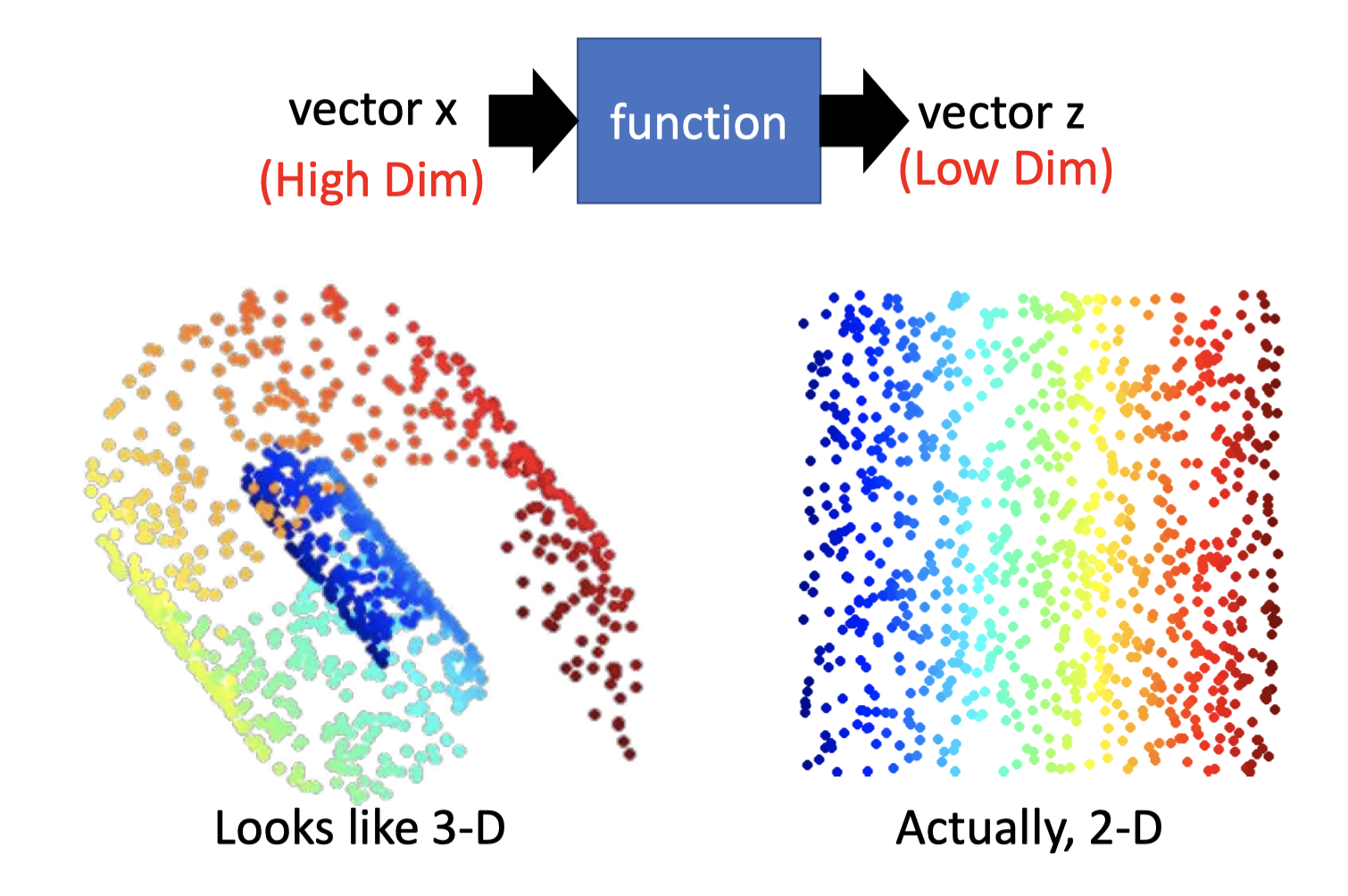• Generation：无中生有。

function 只有output。

（后面的博客会提及）

## Dimension Reduction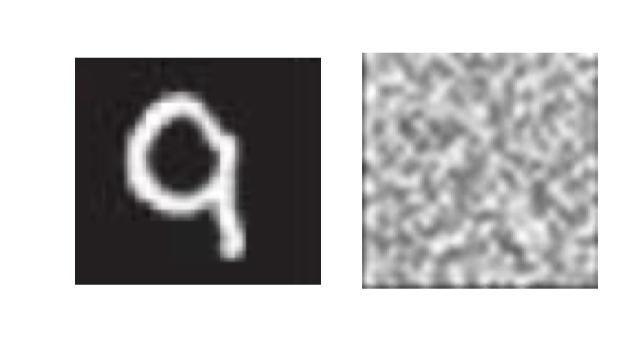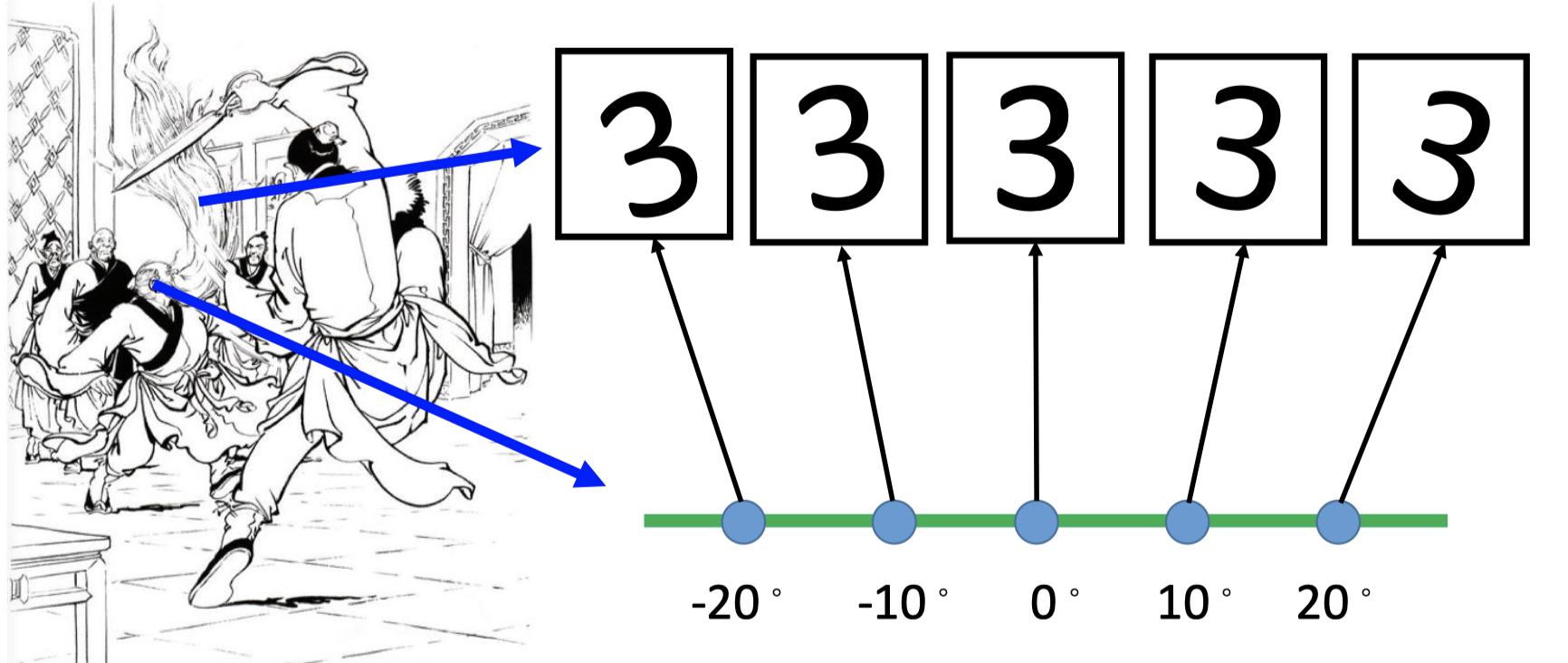## Clustering

clustering也是一种降维的表达，将复杂的向量空间抽象为简单的类别，用某一个类别来表示该数据点。

### K-means

K-means的做法是：

1. Clustering $X=\left\{x^{1}, \cdots, x^{n}, \cdots, x^{N}\right\}$ into K clusters.

把所有data分为K个类，K的确定是empirical的，需要自己确定

2. Initialize cluster center $c^i, i=1,2,…,K$ .(K random $x^n$ from $X$)

初始化K个类的中心数据点，建议从training set $X$ 中随机选K 个点作为初始点。

不建议直接在向量空间中随机初始化K个中心点，因为很可能随机的中心点不属于任何一个cluster。

3. Repeat：根据中心点标记所属类，再更新新的中心点，重复直收敛。

1. For all $x^n$ in $X$ : 标记所属类。

$$b_{i}^{n}\left\{\begin{array}{ll}1 & x^{n} \text { is most "close" to } c^{i} \\ 0 & \text { Otherwise }\end{array}\right.$$
2. Updating all $c^i$ : $c^{i}=\sum_{x^{n}} b_{i}^{n} x^{n} / \sum_{x^{n}} b_{i}^{n}$ (计算该类中心点)

### HAC：Hierarchical Agglomerative Clustering(HAC)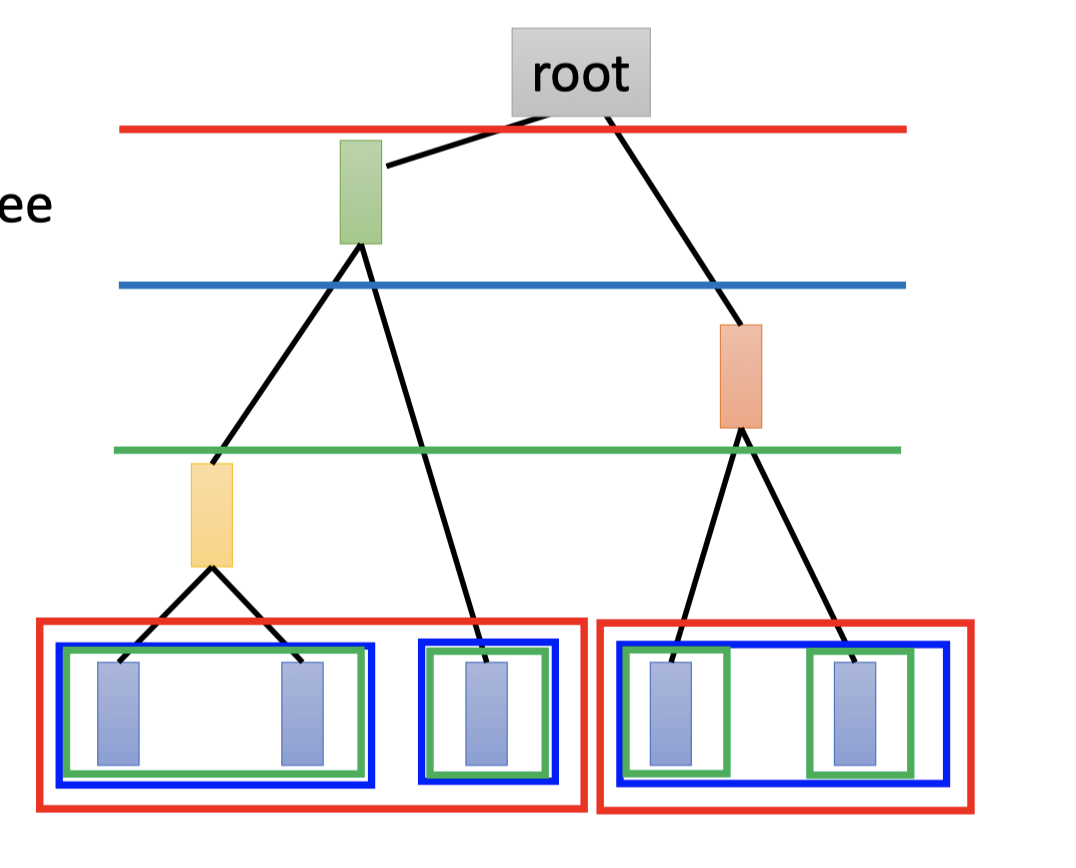1. Build a tree.

1. 如上图中，计算当前两两数据点（点或组合）的相似度（欧几里得距离或其他）。
2. 选出最相近的两个合为一组（即连接在同一父子结点上，如最左边的两个）
3. 重复1-2直至最后合为root。

该树中，越早分支的点集合，说明越不像。

2. Pick a threshold.

选一个阈值，即从哪个地方开始划开，比如选上图中红色的线作为阈值，那么点集分为两个cluseter，蓝色、绿色同理。

HAC和K-means相比，HAC不直接决定cluster的数目，而是通过决定threshold的值间接决定cluster的数目。

## Distributed Representation

Cluster：an object must belong to one cluster.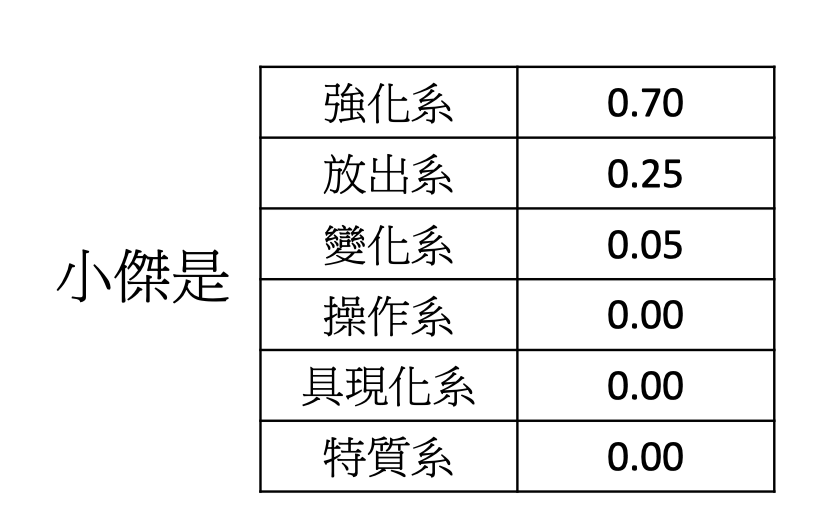Distributed Representation（也叫Dimension Reduction）就是：一个高维的vector通过function，得到一个低维的vector。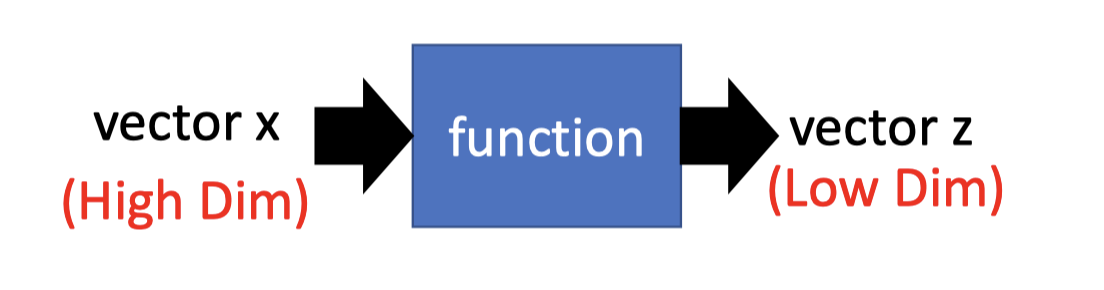Distributed的方法有常见的两种：

• Feature selection：

如下图数据点的分布，可以直接选择feature $x_2$ .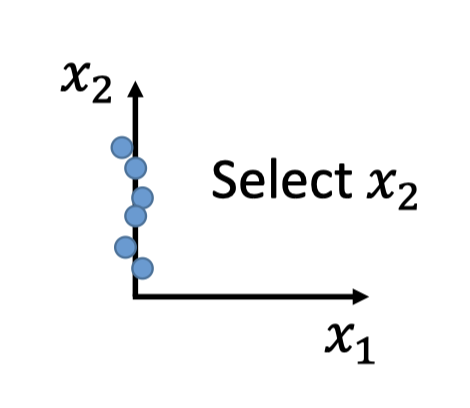但这种方法往往只能处理2-D的情况，对于下图这种3-D情况往往不好做特征选择。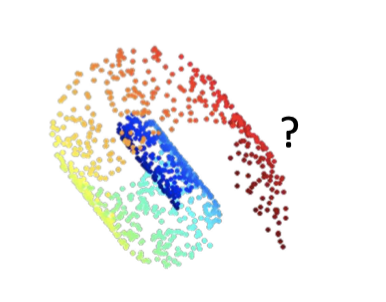• Principle component analysis（PCA）

另一种方法就是著名的PCA，主成分分析法。

PCA中，这个function就是一个简单的linear function（$W$），通过 $z=Wx$ ，将高维的 $x$ 转化为低维的 $z$ .

# PCA：Principle Component Analysis

PCA的参考资料见Bishop, Chapter12.

PCA就是要找 $z=Wx$ 中的 $W$ .

## Main Idea

### Reduce 1-D

$w^1$ 是vector，$x$ 是vector，做内积。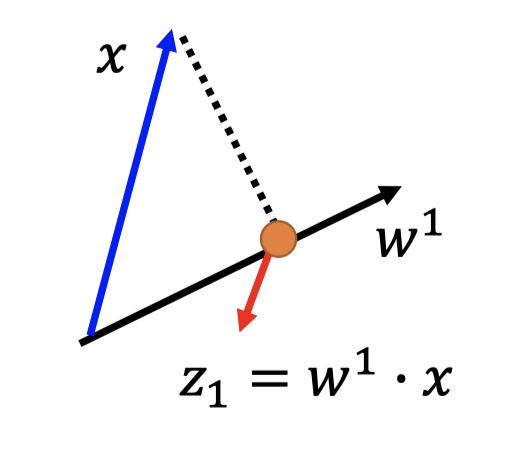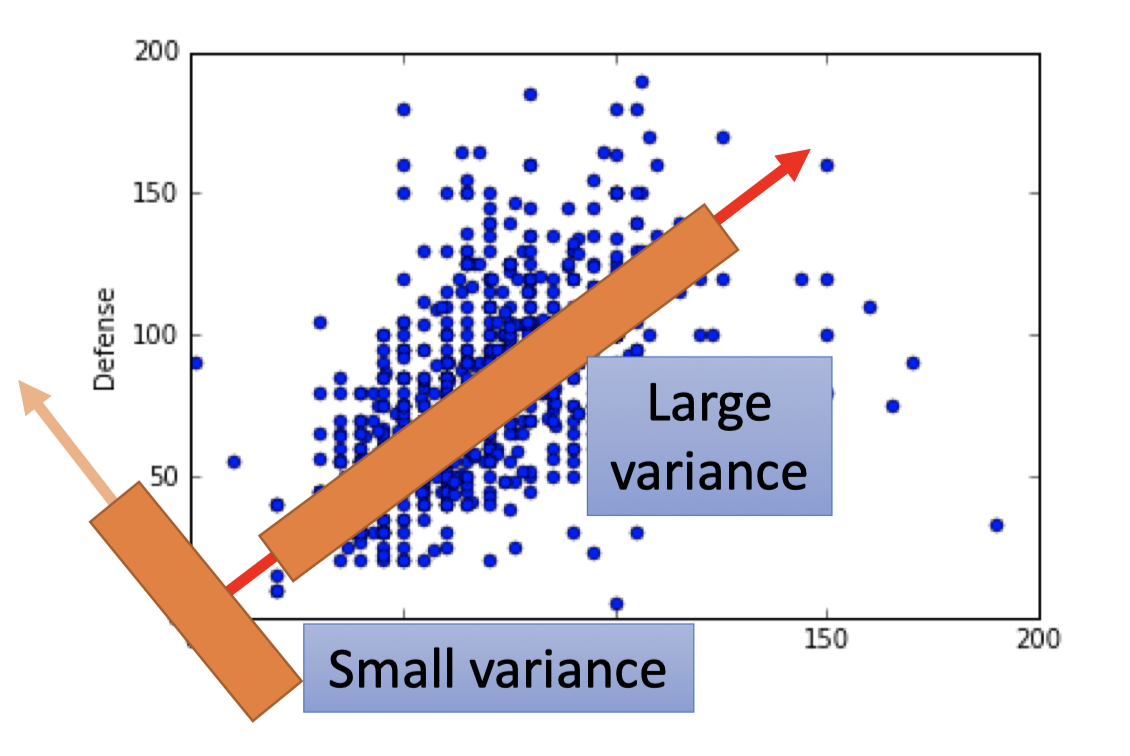$z_1$ 的数学表达是： $\operatorname{Var}\left(z_{1}\right)=\frac{1}{N} \sum_{z_{1}}\left(z_{1}-\overline{z_{1}}\right)^{2} \quad \left\|w^{1}\right\|_{2}=1$ (后文解释为什么要 $w^1$ 的长度为1)

### Reduce 2-D

$z=Wx$ 即

$$\left\{ \begin{array}{11}z_1=w^1\cdot x \\ z_2=w^2 \cdot x \end{array} \right. ,\quad W=\begin{bmatrix}(w^1)^T \\ (w^2)^T \end{bmatrix}$$
• 将所有点 $x$ 投影到 $w^1$ 方向，得到对应的 $z_1$ ，且让 $z_1$ 的分布尽可能的大：

$$\operatorname{Var}\left(z_{1}\right)=\frac{1}{N} \sum_{z_{1}}\left(z_{1}-\overline{z_{1}}\right)^{2} ,\quad \left\|w^{1}\right\|_{2}=1$$
• 将所有点投影到 $w^2$ 方向，得到对应的 $z_2$ ，同样让 $z_2$ 的分布也尽可能大，再加一个约束条件，让 $w^2$ 和 $w^1$ 正交（后文会具体解释为什么）

$$\operatorname{Var}\left(z_{2}\right)=\frac{1}{N} \sum_{z_{2}}\left(z_{2}-\overline{z_{2}}\right)^{2} ,\quad \left\|w^{2}\right\|_{2}=1 ,\quad w^1\cdot w^2=0$$

因此矩阵 $W$ 是Orthogonal matrix (正交矩阵)。

## Detail[Warning of Math

1-D中：

Goal：find $w^1$ to maximum $(w^1)^T S w^1$ s.t.$(w^1)^Tw^1=1$

2-D中：

Goal：find $w^2$ to maximum $(w^2)^TSw^2$ s.t. $(w^2)^Tw^2=1, (w^2)^Tw^1=0$

k-D中：

### 1-D

Goal：Find $w^1$ to maximum the variance of $z_1$ .

• $\operatorname{Var}\left(z_{1}\right)=\frac{1}{N} \sum_{z_{1}}\left(z_{1}-\overline{z_{1}}\right)^{2}$
• $z_1=w^1\cdot x ,\quad \overline{z_{1}}=\frac{1}{N} \sum_{z_{1}}=\frac{1}{N} \sum w^{1} \cdot x=w^{1} \cdot \frac{1}{N} \sum x=w^{1} \cdot \bar{x}$
• $\operatorname{Var}\left(z_{1}\right)=\frac{1}{N} \sum_{z_{1}}\left(z_{1}-\overline{z_{1}}\right)^{2}=(w^1)^T\operatorname{Cov}(x)w^1$
• $=\frac{1}{N} \sum_{x}\left(w^{1} \cdot x-w^{1} \cdot \bar{x}\right)^{2}$
• $=\frac{1}{N} \sum\left(w^{1} \cdot(x-\bar{x})\right)^{2}$
• $a,b$ 是vector：

$(a\cdot b)^2=(a^Tb)^2=a^Tba^Tb$
• $a^Tb$ 是scalar:

$(a\cdot b)^2 = (a^Tb)^2=a^Tba^Tb =a^Tb(a^Tb)^T=a^Tbb^Ta$
• $=\frac{1}{N} \sum\left(w^{1}\right)^{T}(x-\bar{x})(x-\bar{x})^{T} w^{1}$
• $= \left(w^{1}\right)^{T}\sum\frac{1}{N}(x-\bar{x})(x-\bar{x})^{T} \ w^{1}$
• $=(w^1)^T\operatorname{Cov}(x)w^1$
• 令 $S=\operatorname{Cov}(x)$

1. $\left|w^1\right|_2=1$ ?
2. $w^1\cdot w^2=1$ ?

Goal：maximum $(w^1)^T S w^1$ s.t. $(w^1)^Tw^1=1$

• Lagrange multiplier[挖坑] 求解多元变量在有限制条件下的驻点。

构造拉格朗日函数： $g\left(w^{1}\right)=\left(w^{1}\right)^{T} S w^{1}-\alpha\left(\left(w^{1}\right)^{T} w^{1}-1\right)$ ，$\alpha\neq 0$ 为拉格朗日乘数

• $\nabla_{w^1}g=0$ 的值为驻点（会单独写一篇博客来讲拉格朗日乘数）
• $\frac{\partial g}{\partial \alpha}=0$ 为限制函数
• 对矩阵微分：详情见wiki

• scalar-by-vector(scalar对vector微分)• $S$ 是对称矩阵，不是 $w^1$ 的函数，结果用 $w^1$ 表达：$2Sw^1-2\alpha w^1=0$

• maximum: $(w^1)^T S w^1=\alpha (w^1)^Tw^1=\alpha$

*Goal：find $w^1$to maximum $\alpha$ *

• $\alpha$ 满足等式：$Sw^1=\alpha w^1$

• $\alpha$ 是 $S$ 的特征向量，$w^1$ 是 $S$ 对应于特征值 $\alpha$ 的特征向量。

• 关于特征值和特征向量的知识参考：参考下面线代知识
• $w^1$ is the eigenvector(特征向量) of the covarivance matrix S corresponding to the largest eigenvalue $\lambda_1$ .

结论：$w^1$ 就是协方差矩阵最大特征值对应的特征向量。

1. 特征向量，特征值定义：

$A$ 是n阶方阵，如果存在数 $\lambda$ 和n维非零向量 $\alpha$ ，满足 $A\alpha=\lambda \alpha$ ,

则称 $\lambda$ 为方阵 $A$ 的一个特征值，$\alpha$ 为方阵 $A$ 对应于特征值 $\lambda$ 的一个特征向量。

2. 求解特征向量和特征值：

$A\alpha -\lambda \alpha=(A-\lambda I)\alpha=0$

齐次方程有非零解的充要条件是特征方程 $det(A-\lambda I)=0$ （行列式为0）

• 根据特征方程先求解出 $\lambda$ 的所有值。
• 再根将 $\lambda$ 代入齐次方程，求解齐次方程的解 $\alpha$ ，即为对应 $\lambda$ 的特征向量。

### 2-D

Goal：find $w^2$ to maximum $(w^2)^TSw^2$ s.t. $(w^2)^Tw^2=1, (w^2)^Tw^1=0$

• 构造拉格朗日函数： $g\left(w^{2}\right)=\left(w^{2}\right)^{T} S w^{2}-\alpha\left(\left(w^{2}\right)^{T} w^{2}-1\right)-\beta\left(\left(w^{2}\right)^{T} w^{1}-0\right)$
• 对 $w^2$ 求微分，所求点满足等式： $S w^{2}-\alpha w^{2}-\beta w^{1}=0$
• 左乘 $(w^1) ^T$： $(w^1)^TSw^2-\alpha (w^1)^Tw^2-\beta(w^1)^Tw^1=0$
• 已有： $(w^1)^Tw^2=0, (w^1)^Tw^1=1$
• 证明：$(w^1)^TSw^2=0$
• $\because (w^1)^TSw^2$ 是scalar
• $\therefore (w^1)^TSw^2=((w^1)^TSw^2)^T=(w^2)^TS^Tw^1$
• $\because S^T=S$ (协方差矩阵是对称矩阵)
• $\because Sw^1=\lambda_1 w^1$
• $\therefore (w^1)^TSw^2=(w^2)^TSw^1=\lambda_1(w^2)^Tw^1=0$
• $(w^1)^TSw^2-\alpha (w^1)^Tw^2-\beta(w^1)^Tw^1=0-\alpha\cdot 0-\beta \cdot 1=0$
• $\therefore \beta=0$
• $w^2$ 满足等式：$S w^{2}-\alpha w^{2}=0$
• 和1-D的情况相同：find $w^2$ maximum $(w^2)^TSw^2$
• $(w^2)^TSw^2=\alpha$
• $w^2$ is the eigenvector(特征向量) of the covarivance matrix S corresponding to the largest eigenvalue $\lambda_2$ .
• OVER!

### Conclusion

• 先说明一下$S$ 的性质：

是对称矩阵，对应不同特征值对应的特征向量都是正交的。

（参考1，2）

也是半正定矩阵，其特征值都是非负的。

（参考4，5，6）

• 其次关于 $W$ 的性质 $W=\begin{bmatrix}(w^1)^T \\ (w^2)^T \\ ...\end{bmatrix}$ ,易得 $W$ 是orthogonal matrix(正交矩阵)。

• 所以这是一个约束条件，能让PCA的最优化问题转化为求其特征值的问题。

• （具体见下一小节：PCA-decorrelation）

其次 $z=Wx$ ，也因为 $W$ 的正交性质，让 $z$ 的各维度（特征）decorrelation，去掉相关性，降维后的特征相互独立，方便后面generative model的假设。

1. $S=Cov(x)$ 为实对称矩阵。

2. 实对称矩阵的性质：$A$ 是一个实对称矩阵，对于于 $A$ 的不同特征值的特征向量彼此正交。

3. 正交矩阵的性质：$W^TW=WW^T=I$

4. $Var(z)=(w^1)^T S w^1\geq 0$ ，方差一定大于等于0 。

5. 半正定矩阵的定义：

实对称矩阵 $A$ ，对任意非零实向量 $X$ ，如果二次型 $f(X)=X^TAX\geq0$ ，

则有实对称矩阵 $A$ 是半正定矩阵。

6. 半正定矩阵的性质：半正定矩阵的特征值都是非负的。

1-D中：

Goal：find $w^1$ to maximum $(w^1)^T S w^1$ s.t.$(w^1)^Tw^1=1$

2-D中：

Goal：find $w^2$ to maximum $(w^2)^TSw^2$ s.t. $(w^2)^Tw^2=1, (w^2)^Tw^1=0$

k-D中：

## PCA-decorrelation

$z=Wx$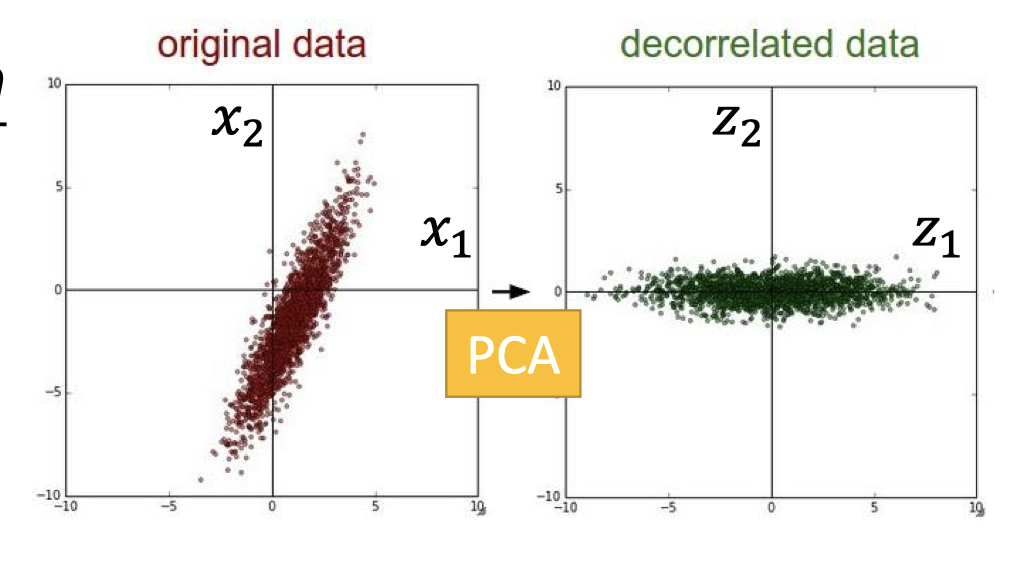$z$ 是docorrelated，即 $Cov(z)=D$ 是diagonal matrix(对角矩阵)

• $W=\begin{bmatrix}(w^1)^T \\ (w^2)^T \\ ...\end{bmatrix}$ ，$S=\operatorname{Cov}(x)$
• $\operatorname{Cov}(z)=\frac{1}{N} \sum(z-\bar{z})(z-\bar{z})^{T}=W S W^{T}$
• $=W S\left[\begin{array}{lll}w^{1} & \cdots & w^{K}\end{array}\right]=W\left[\begin{array}{lll}S{w}^{1} & \cdots & S w^{K}\end{array}\right]$
• $=W\left[\lambda_{1} w^{1} \quad \cdots \quad \lambda_{K} w^{K}\right]=\left[\lambda_{1} W w^{1} \quad \cdots \quad \lambda_{K} W w^{K}\right]$ ($\lambda$ is scalar)
• $=\left[\begin{array}{lll}\lambda_{1} e_{1} & \cdots & \lambda_{K} e_{K}\end{array}\right]=D$ ($W$ is orthogonal matrix)

## PCA-Another Point of View

### Main Idea: Component

PCA看作是一些basic component的组成，如下图，手写数字都是一些基本笔画组成的，记做 $\{u^1,u^2,u^3,...\}$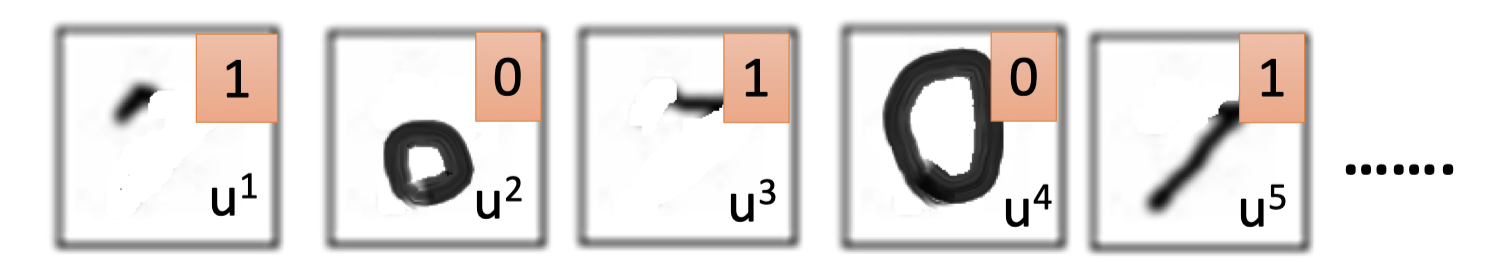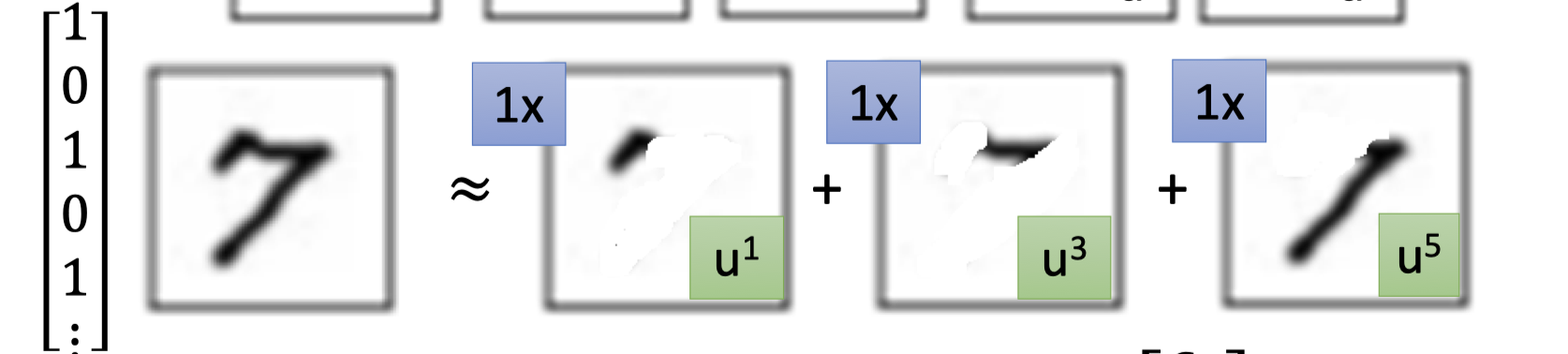$$x \approx c_{1} u^{1}+c_{2} u^{2}+\cdots+c_{K} u^{K}+\bar{x}$$

### Detail

*Goal: minimize the reconstruction error = $\|(x-\bar{x})-\hat{x}\|_2$ *

• $x -\bar{x}\approx c_{1} u^{1}+c_{2} u^{2}+\cdots+c_{K} u^{K}$

• 每个sample: $\left\{ \begin{matrix} x^{1}-\bar{x} \approx c_{1}^{1} u^{1}+c_{2}^{1} u^{2}+\cdots \\ x^{2}-\bar{x} \approx c_{1}^{2} u^{1}+c_{2}^{2} u^{2}+\cdots \\x^{3}-\bar{x} \approx c_{1}^{3} u^{1}+c_{2}^{3} u^{2}+\cdots \\ ...\end{matrix} \right.$

• 下图中 $X=x-\bar{x}$ 矩阵的第一列都和上面的 $x^1-\bar{x}$ 对应：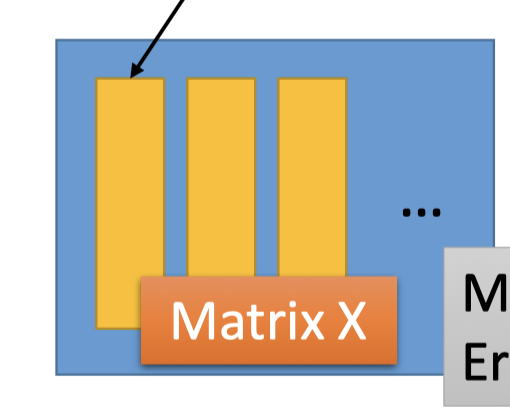• 而上面的 $c_{1}^{1} u^{1}+c_{2}^{1} u^{2}+\cdots$ 和下图的component矩阵乘系数矩阵的第一列对应：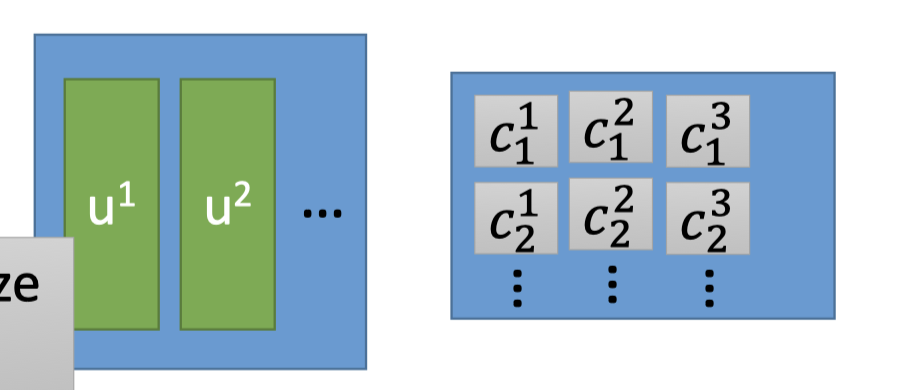• 因此，是要让下图矩阵的结果 minimize error：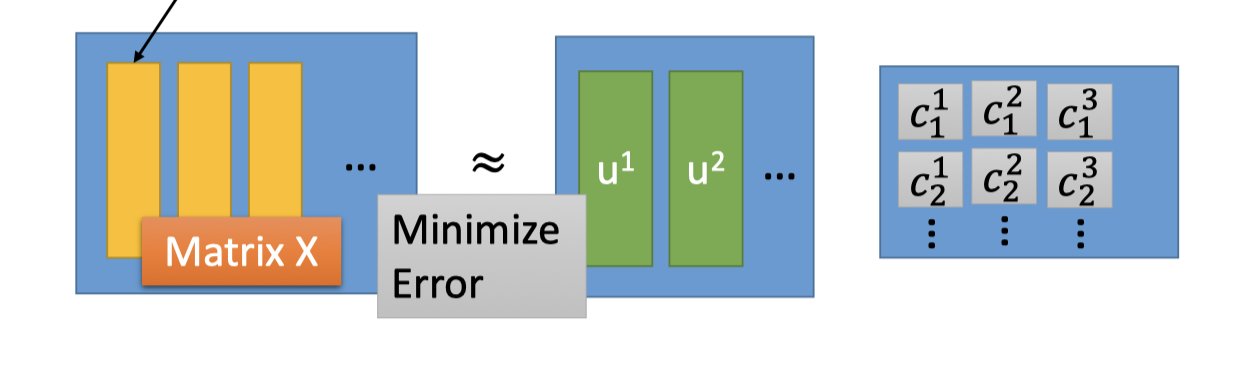• 如何求解: SVD矩阵分解-其实就是最大近似分解（挖坑）

SVD能将一个任意的矩阵，分解为下面三个矩阵的乘积。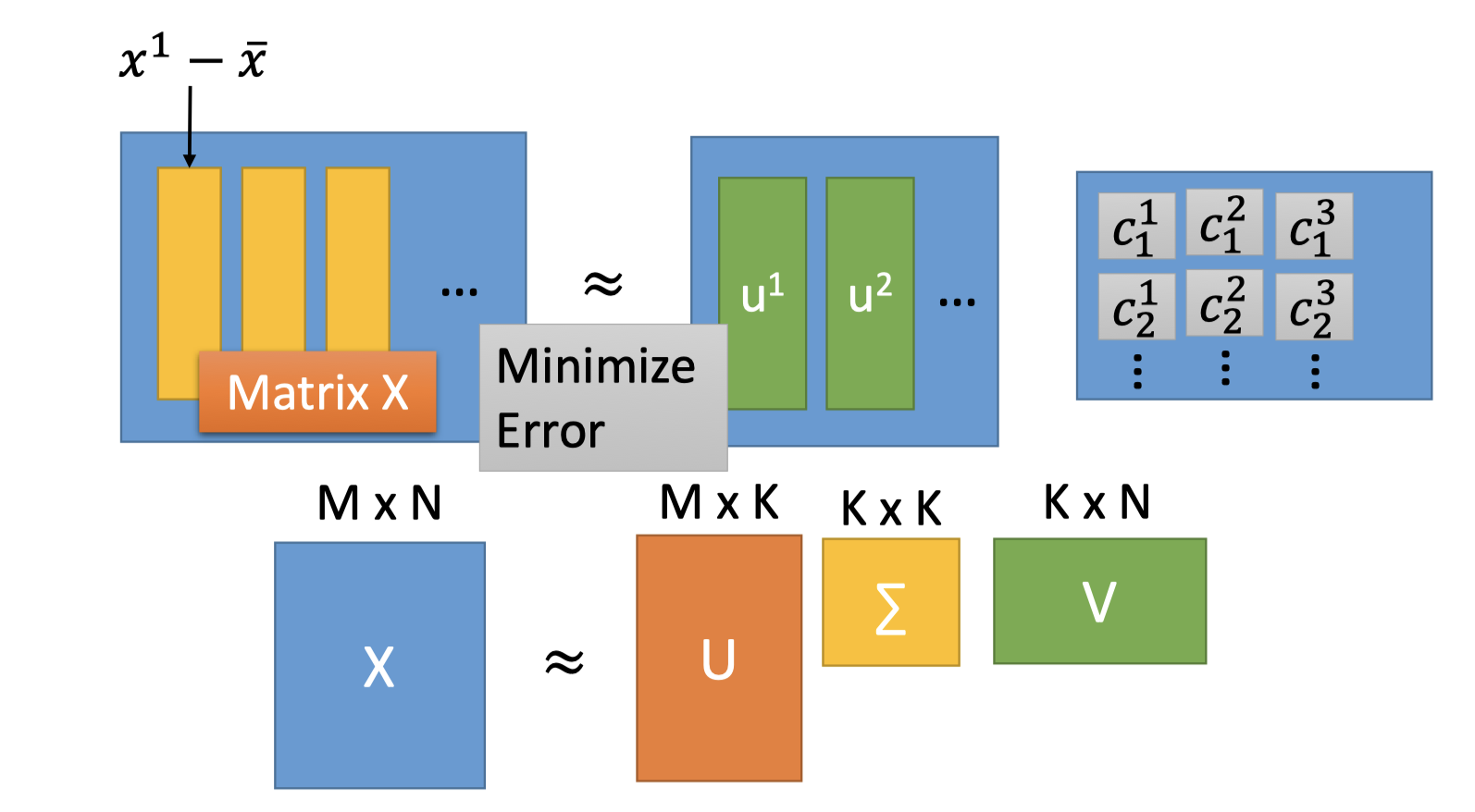$X = U\Sigma V$

• $U,V$ 都是orthogonal matrix，$\Sigma$ 是diagonal matrix。
• 组成$U$ (M*K) 的K个列向量是 $XX^T$ 矩阵的前K大特征值对应的特征向量。
• 组成 $V$ (K*N)的K个行向量是 $X^TX$ 矩阵的前K大特征值对应的特征向量。
• $XX^T$ 和 $X^TX$ 的特征值相同
• $\Sigma$ 的对角值 $\sigma_i=\sqrt{\lambda_i}$
• 解：$U$ 矩阵作为 component矩阵， $\Sigma V$ 乘在一起作为系数矩阵。

$U=\{u^1,u^2,u^3,...\}$ 矩阵是$XX^T$ 的特征向量组成正交矩阵。

### PCA-NN：Autoencoder

PCA视角： $z=c_k=(x-\bar{x})\cdot w^k$

PCA looks like a neural network with one hidden layer(linear activation function)。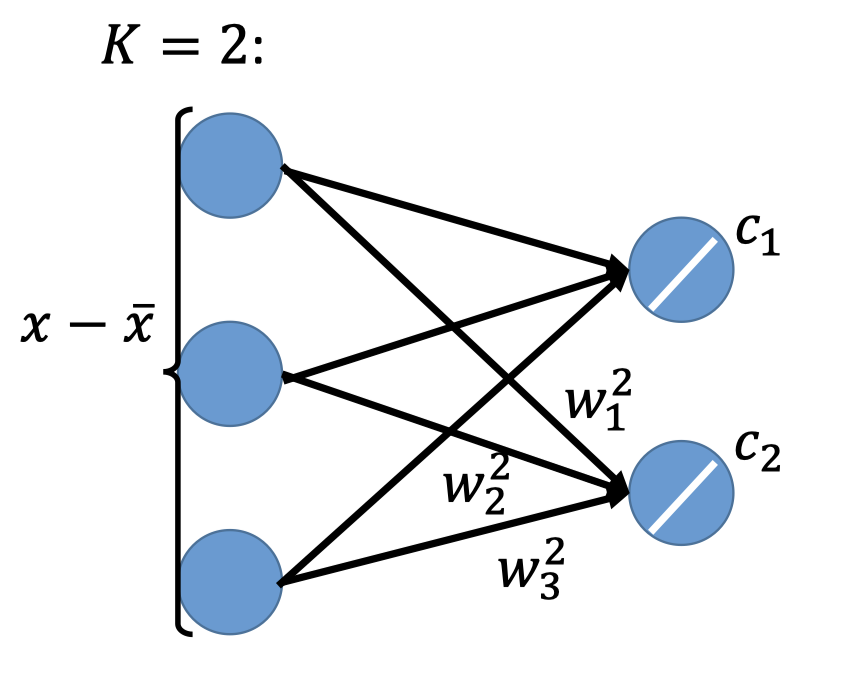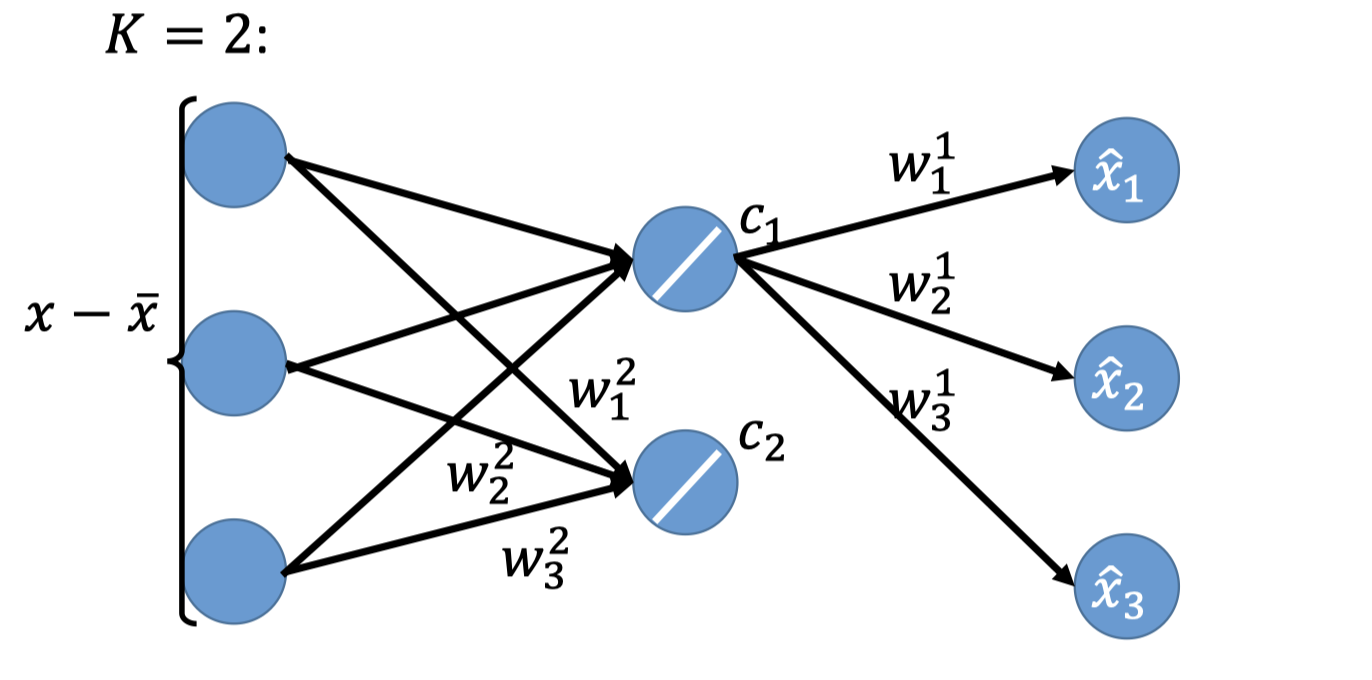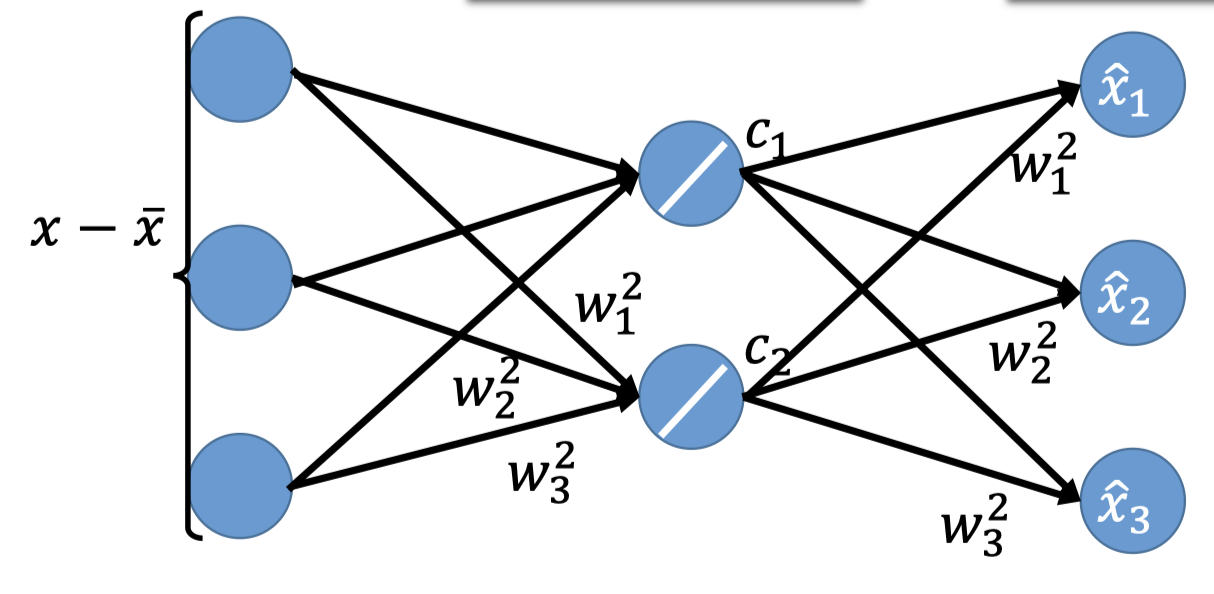PCA就构成了下面的NN，hidden layer可以是deep，这就是autoencoder(后面的博客会再详细讲)。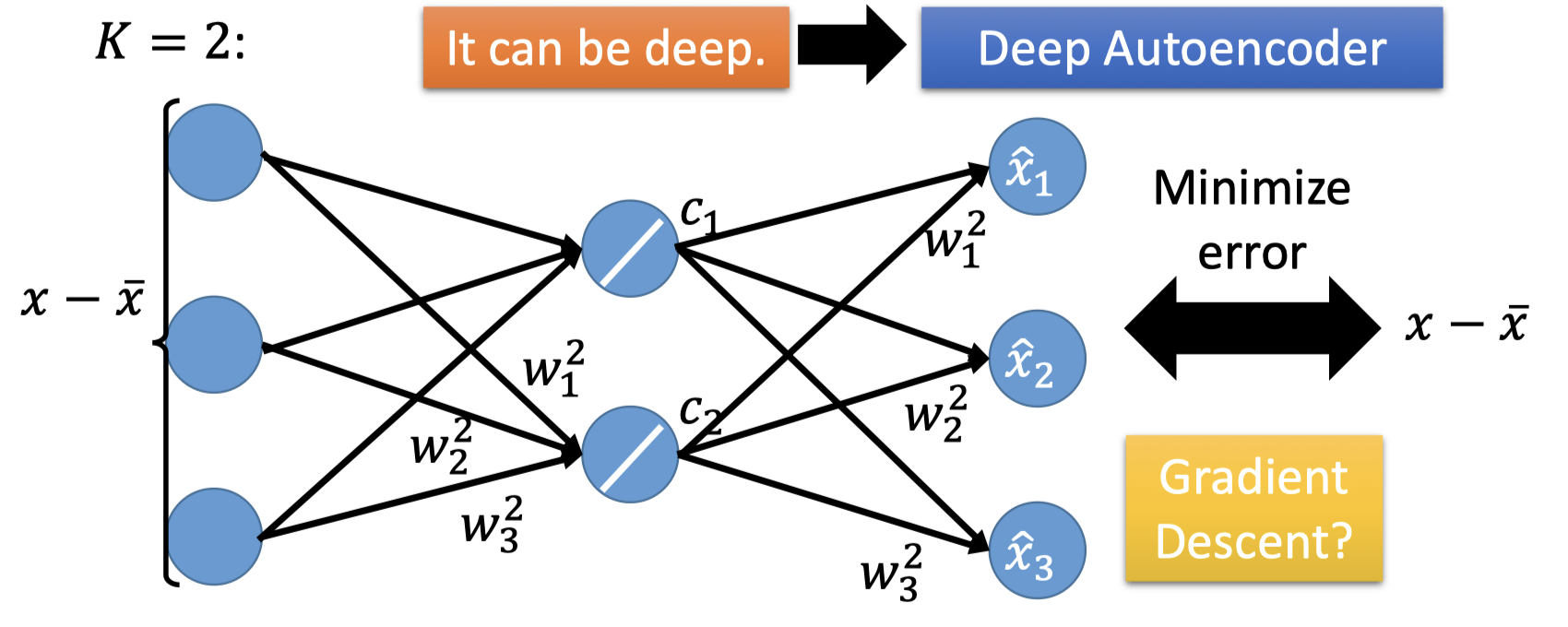A：当然不一样，PCA的 $w$ 都是正交的，而NN的结果是gradient descent迭代出来的，并且该结果还会于初值有关。

Q：有了PCA，为什么还要用NN呢？

A：因为PCA只能处理linear的情况，对前文那种高维的非线形的无法处理，而NN可以是deep的，能较好处理非线形的情况。

## tips: how many components?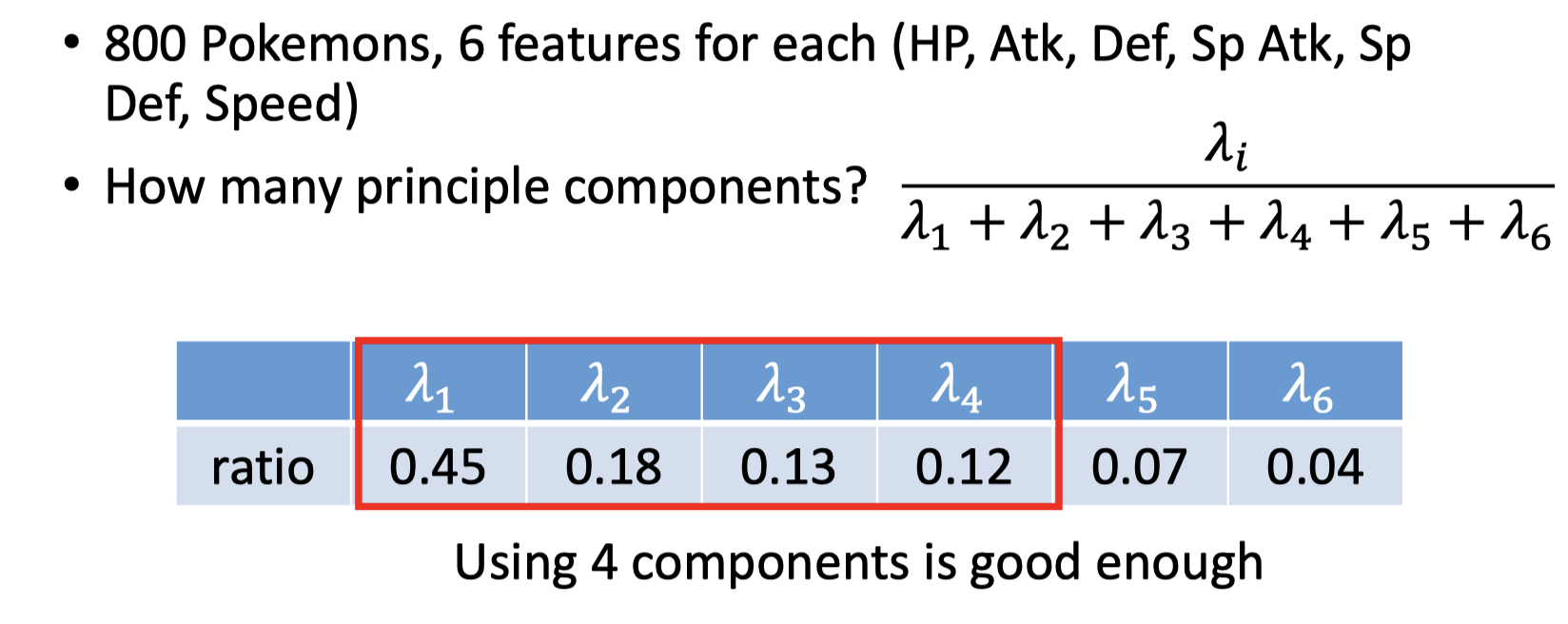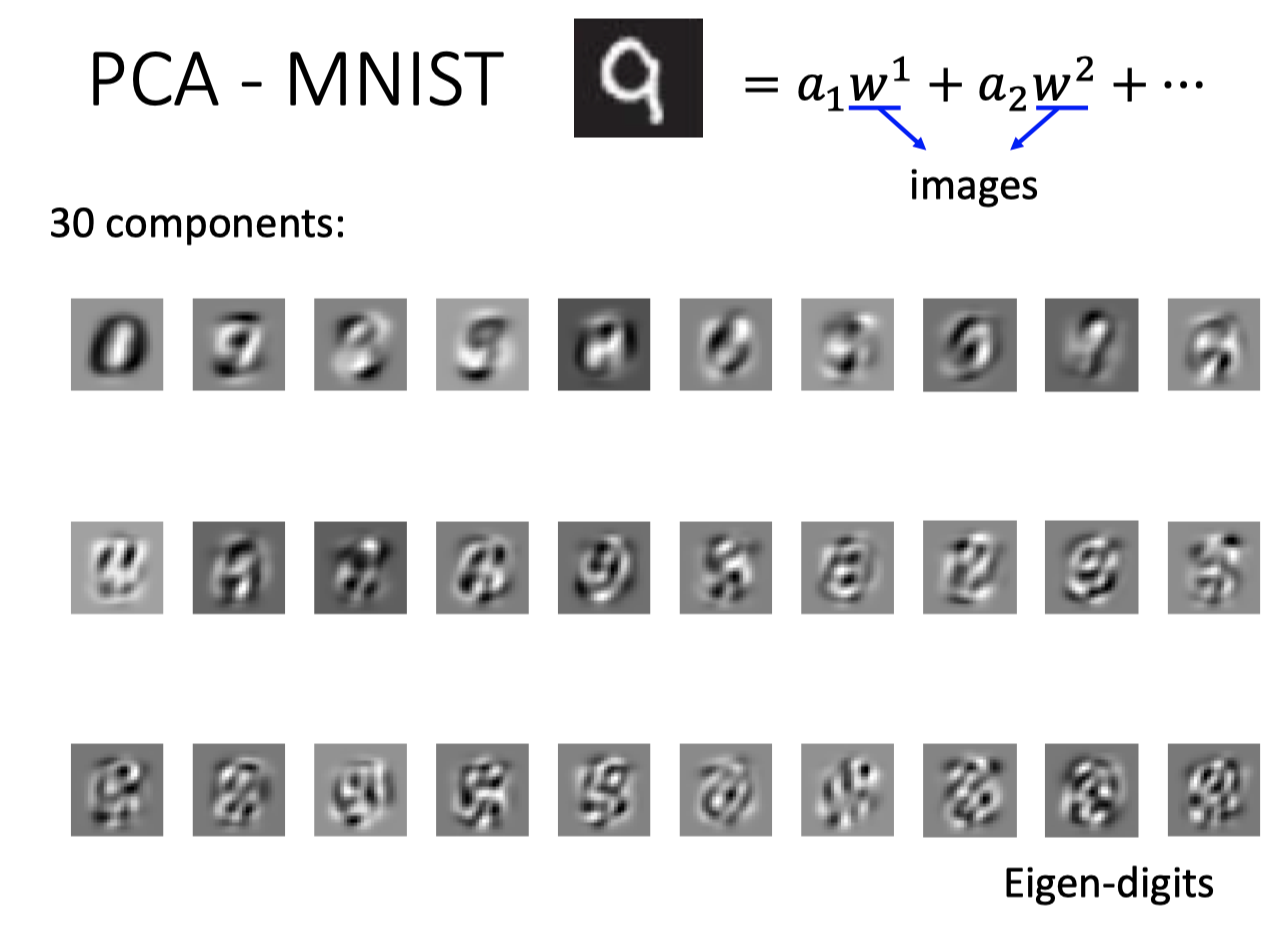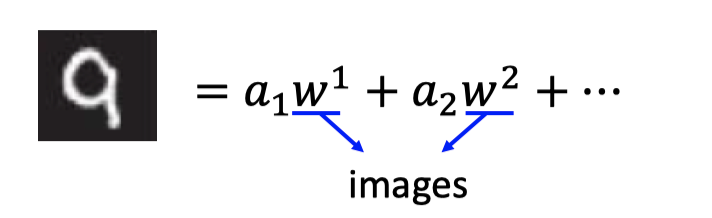• Non-negative matrix factorization(NMF)
• Forcing $\alpha$ be non-negative: additive combination
• Forcing $w$ be non-negative: components more like “parts of digits”

## Weakness of PCA

1. PCA是unsupervised，因此可能不能区分本来是两个类别的东西。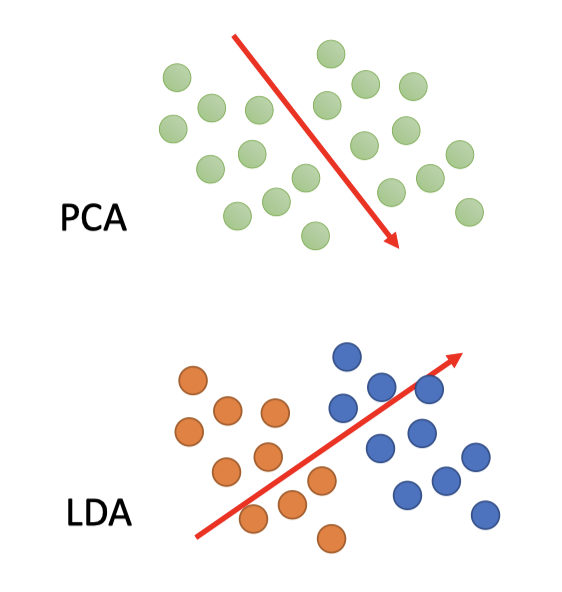如图，PCA的结果可能是上图的维度方向，但如果引入labeled data，更好的表达应该按照下图LDA的维度方向。

• LDA (Linear Discriminant Analysis) 是一种supervised的分析方法。
2. PCA是Linear的，前文已经提及过，除了可以用NN的方式也有很多其他的non-linear的解法。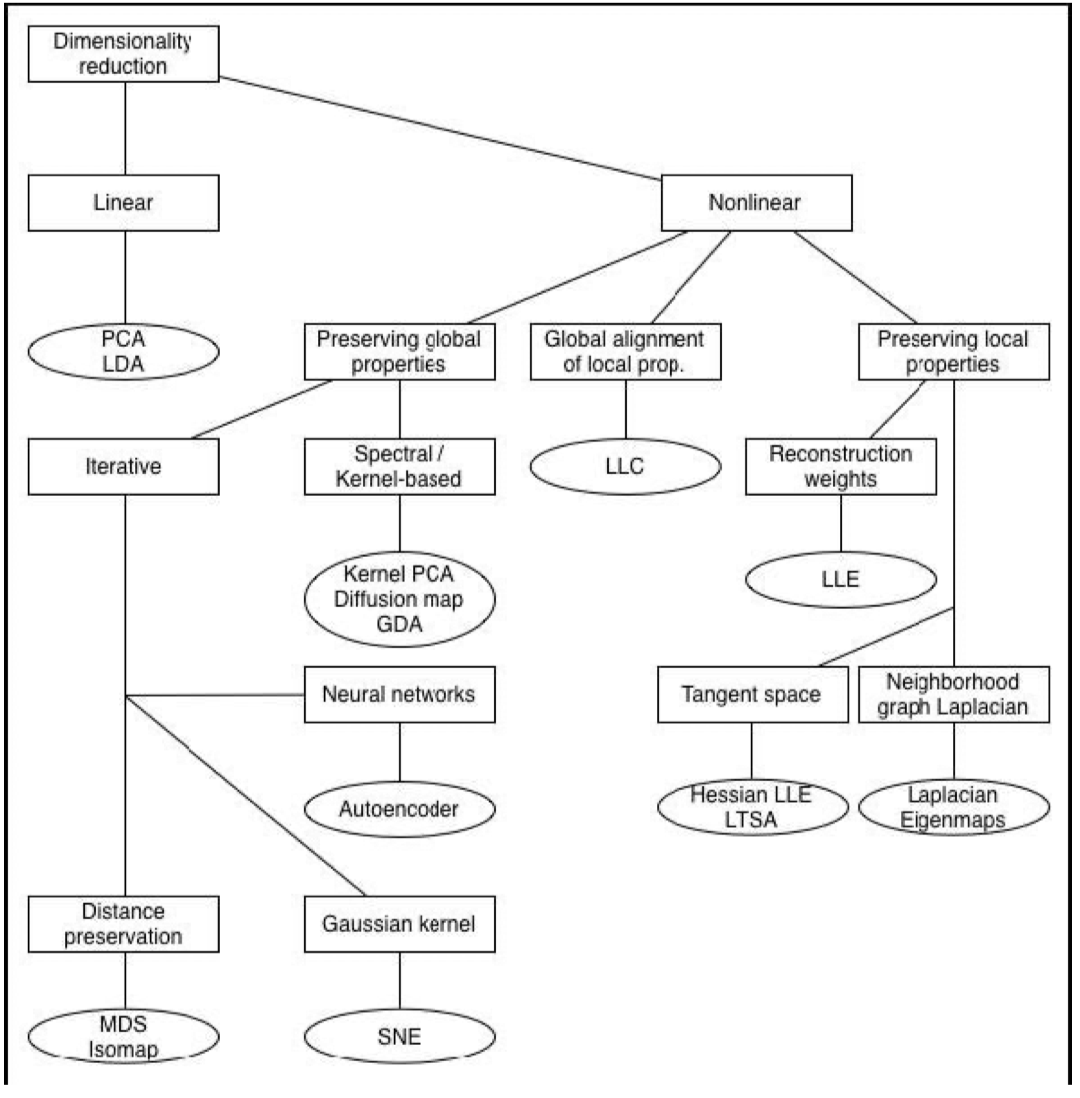# Reference

1. HAC的算法细节待补充完善：https://zhuanlan.zhihu.com/p/34168766

2. PCA: Bishop, Chapter12.

3. 线代知识：特征值、特征向量、实对称矩阵等：

4. 拉格朗日乘数：Bishop, Appendix E

5. Proof-PCA的过程就是在minimize损失函数 $L$ :Bisho, Chapter 12.1.2

6. SVD：